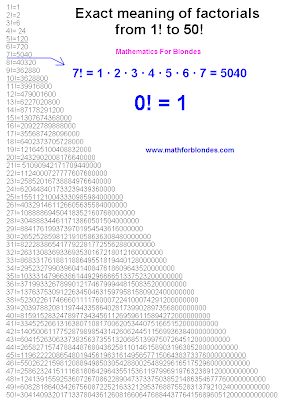## 2/08/2011

### Factorial

Factorial in the mathematician name product of all natural numbers, including the specified number. The factorial by an exclamation mark after number, for example 4 is designated!. So, if you have met an exclamation mark in the mathematician, it at all does not mean "Vau! Number!". It simply factorial. From sacred mathematical texts it is necessary to learn one phrase "a zero Factorial it is equal to unit". Why the zero factorial is equal to unit? Read under the reference. Exact values of factorials of numbers to 50 are resulted in drawing. You can copy the exact numerical values of the factorial on this page. You can copy the exact numerical values of the factorial on this page.Factorial
On a picture it is shown how to consider a factorial for natural number 7. Calculation of a factorial of other numbers is made in the same way: all numbers from one to specified before an exclamation mark are multiplied among themselves.

The factorial of 1 (unit) is equal to unit.
1! = 1
The factorial of 2 (two) is equal to two.
2! = 1 · 2 = 2
The factorial of 3 (three) is equal six.
3! = 1 · 2 · 3 = 6
The factorial of 4 (four) equals to twenty four.
4! = 1 · 2 · 3 · 4 = 24
The factorial 5 is equal hundred twenty.
5! = 1 · 2 · 3 · 4 · 5 = 120
Well and so on.

In a general view the formula for a factorial finding can be written down so:

n! = 1 · 2 · 3 · 4 · ... · (n - 2) · (n - 1) · n

The table of factorials to 255 is presented on separate page.

By the way, if you go at the wheel the car and will see an exclamation mark in a triangle on a white or yellow background is not a lesson of mathematics with factorials, it is a traffic sign "Attention!".Factorial )))
Here it is not necessary anything the friend on the friend to multiply. It is necessary to postpone away the beautician, to cease to stir by a mobile phone and more strong to keep for a car wheel. Attentively look not on the parties, and at road. Ahead there can be unpleasant surprises. That unpleasant surprises on road did not turn to unpleasant situations, them designate this traffic sign.

Table of primes 2 to 20,000.